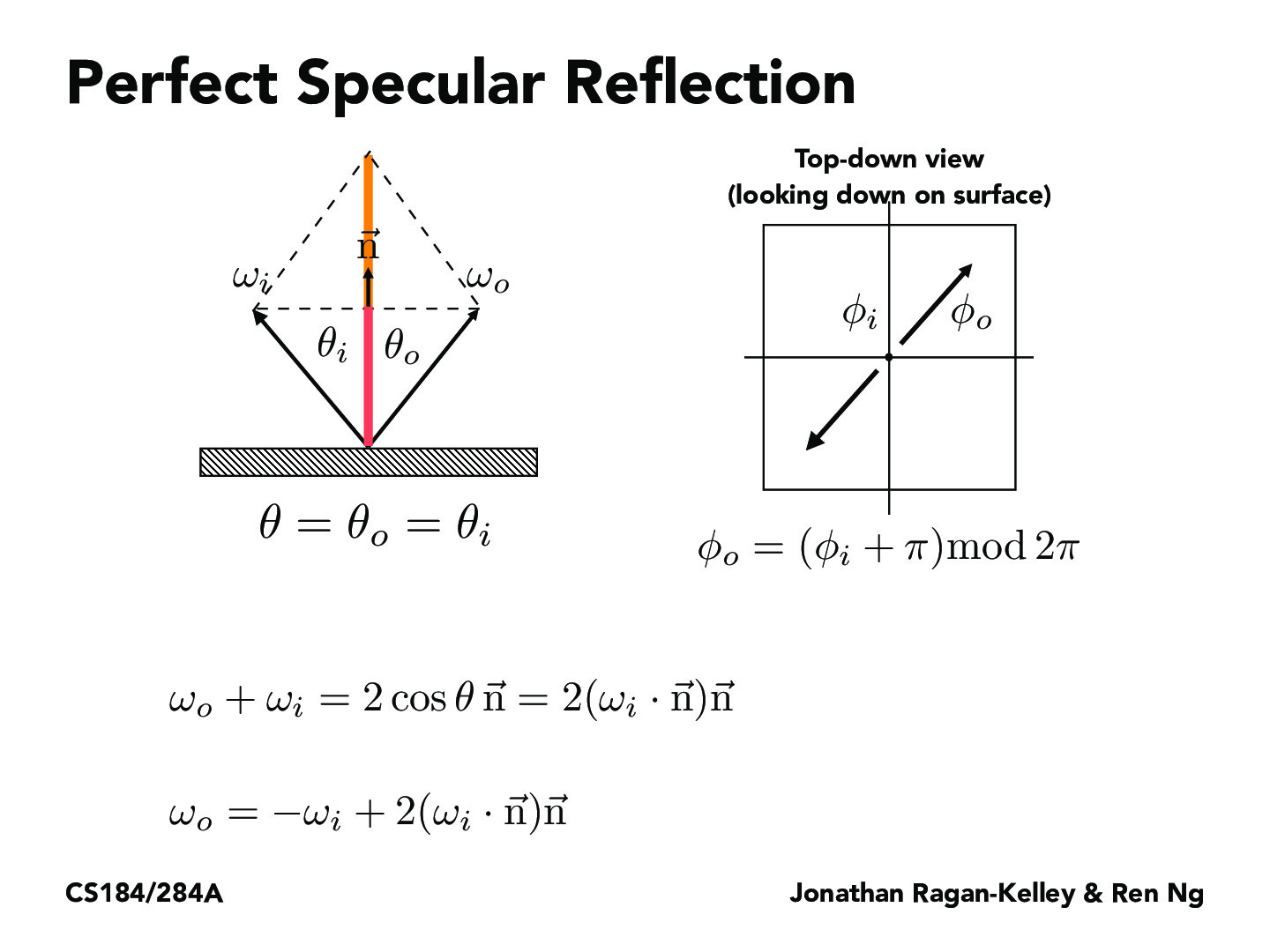You are viewing the course site for a past offering of this course. The current offering may be found here.Lecture 14: Material Modeling (16)emilyzhong

Is there a reason why w_i is pointed outwards away from the surface as opposed to pointed towards the surface — is it just so that the equation is easier to understand/comprehend? I thought w_i, being the incoming ray, should be pointed towards the incident surfacefywu85

$w_i$ and $w_o$ are simply the two distinct equal-length outward-pointing vectors along the path of a reflected light. The point of the left diagram is to show that a perfect reflection will satisfy $w_o = -w_i + 2(w_i \cdot \vec{n})\vec{n}$. I can see why this could be confusion since lights would travel in the direction of $w_i$ and $-w_o$ (or $-w_i$ and $w_o$).jenzou

To emilyzhong, fywu85: I believe $w_i$ points outwards away from the surface so that $cos \theta$ is equal to the dot product between $w_i$ and $\vec{n}$. If it pointed towards the surface, then we can get the same result by replacing $w_i$ with $-w_i$.

You must be enrolled in the course to comment